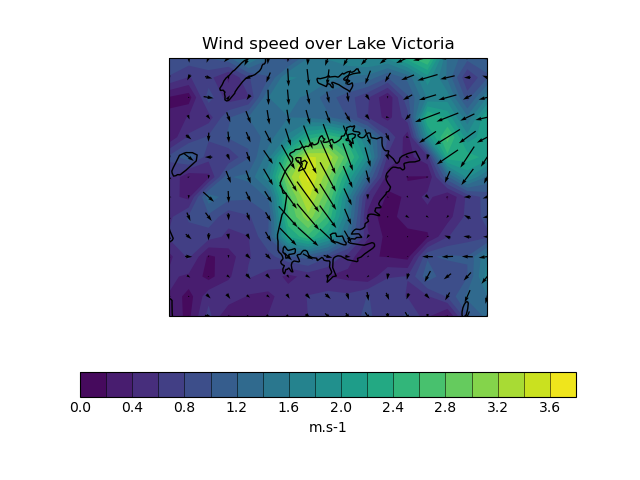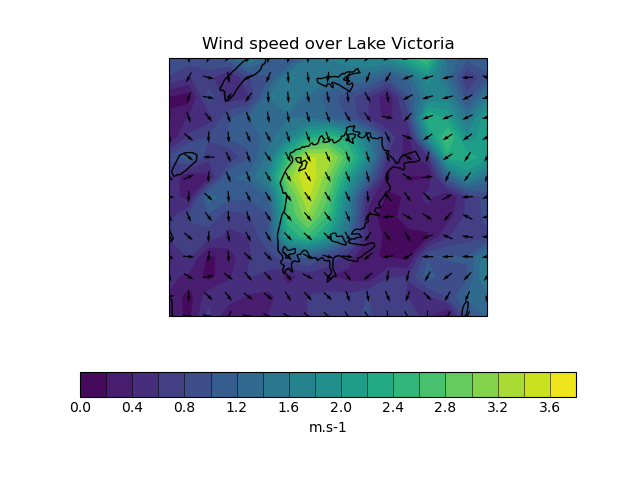# Plotting Wind Direction Using Quiver#

This example demonstrates using quiver to plot wind speed contours and wind direction arrows from wind vector component input data. The vector components are co-located in space in this case.

For the second plot, the data used for the arrows is normalised to produce arrows with a uniform size on the plot.

••```import cartopy.feature as cfeat
import matplotlib.pyplot as plt

import iris
import iris.plot as iplt
import iris.quickplot as qplt

def main():
# Load the u and v components of wind from a pp file.
infile = iris.sample_data_path("wind_speed_lake_victoria.pp")

# Create a cube containing the wind speed.
windspeed = (uwind**2 + vwind**2) ** 0.5
windspeed.rename("windspeed")

# Plot the wind speed as a contour plot.
qplt.contourf(windspeed, 20)

# Show the lake on the current axes.
lakes = cfeat.NaturalEarthFeature(
"physical", "lakes", "50m", facecolor="none"
)

# Add arrows to show the wind vectors.
iplt.quiver(uwind, vwind, pivot="middle")

plt.title("Wind speed over Lake Victoria")
qplt.show()

# Normalise the data for uniform arrow size.
u_norm = uwind / windspeed
v_norm = vwind / windspeed

# Make a new figure for the normalised plot.
plt.figure()

qplt.contourf(windspeed, 20)